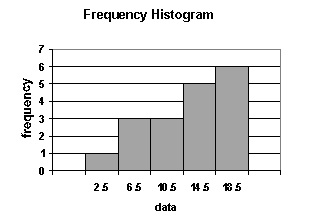### CFA Practice Question

There are 985 practice questions for this topic.

### CFA Practice Question

Use the following frequency histogram to answer this question:Determine the shape of the distribution shown in the histogram.

A. uniform
B. skewed left
C. skewed right

User Comment
leoo why?
cfaman you should be able to tell from the shape of the distribution!
chuong Look at the gray shape of histogram and guess
Yooo Mode: 18.5
Mean: <18.5; Pulled down by 2.5 and 6.5
Rotigga Don't just guess unless you want to fail Level I. Write out the values and frequencies:

Value, Frequency
----------------
2.5, 1
6.5, 3
10.5, 3
14.5, 5
18.5, 6
=======
18

See that the Median=14.5, while the Mean=10.5; since Mean < Median, the distribution is Skewed Left.
Lucho mean 13.17
fahad why is this skewed left? Can anyone explain? How do you get 14.5 as median and 10.5 as mean?
guai Median: 18 scores in total, the average value of the 9th & 10th scores is the median, which is (14.5+14.5)/2=14.5

Mean : (2.5x1+6.5x3+10.5x3+14.5x5+18.5x6)/18=13.17

The mean 13.17 is less than the median 14.5, the distributions is skewed left.
surob Guai - good explanation. I appreciate it.
TammTamm If we look at the shape of the distribution, it clearly shows it's skewed to the left. No calculations required.
magicchip What is there to calculate? the shape is right in front of you...
Sabs Why Calculate? Most of the values (shown by frequency) are to the right, therefore we can safely say that lower values are the long tails. This means negative or skewed to the left.
rfvo Come on...easy. Just look at the shape....For newbies ignore Rotigga comment on working it out first...this should take you 1sec to fiqure out. You dont have time to work things out in the exam. 1.5 min per question. You do the math
jonan203 the side which get screwed, is the side which is skewed...
johntan1979 Yup, agree on ignoring Rotigga's comment. No time to do stuff like that in the actual exam. Learn the shape and this should not take more than a few seconds.
sgossett86 look at it as the tail is longer on the left.. negative left skew
Af00913 Mean is less than mode, left skew